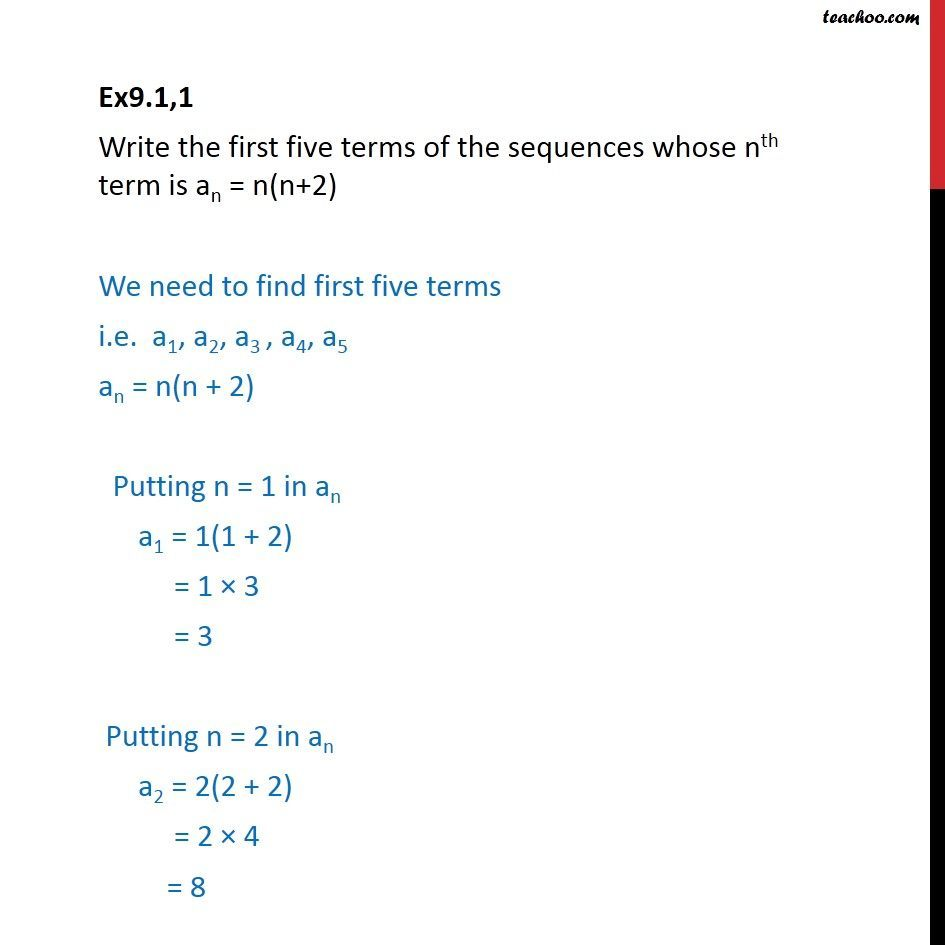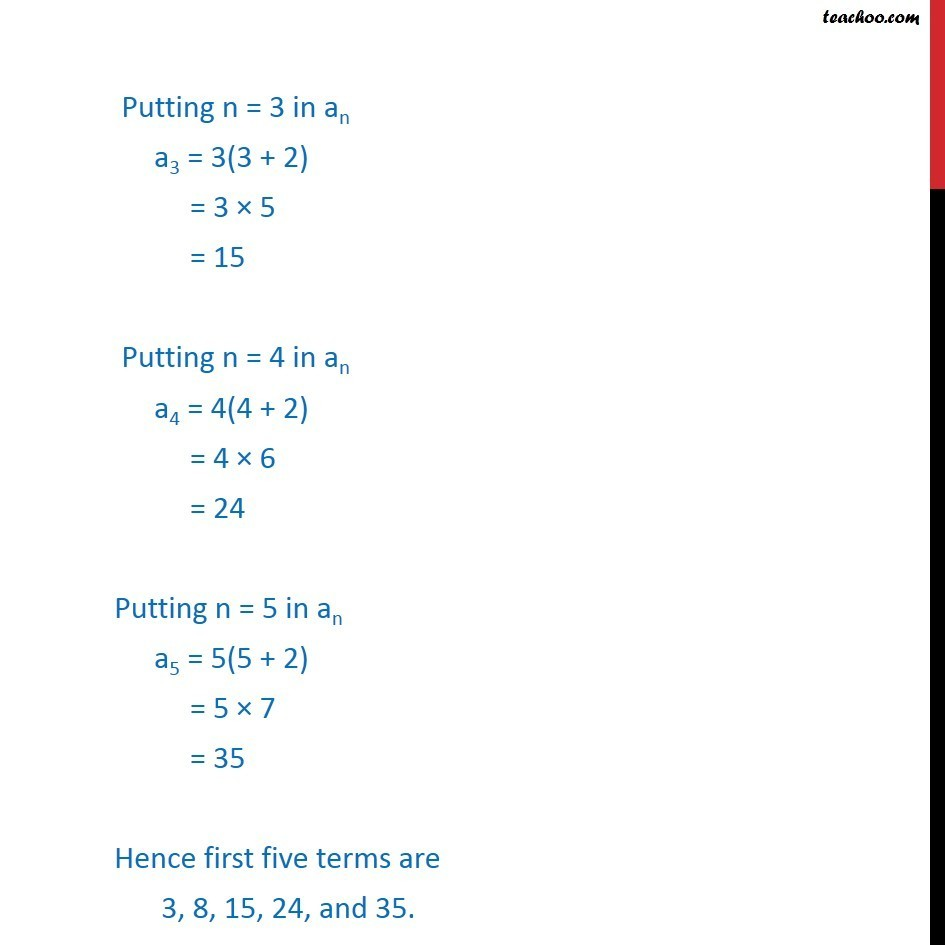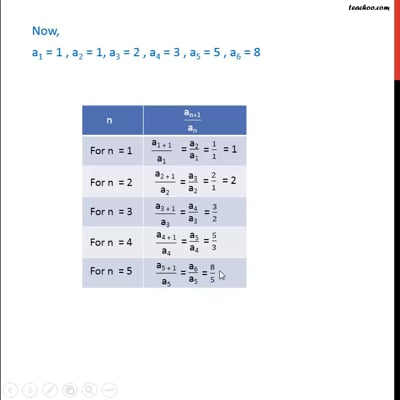Finding Sequences

Chapter 9 Class 11 Sequences and Series
Concept wiseThis video is only available for Teachoo black users

Introducing your new favourite teacher - Teachoo Black, at only ₹83 per month

### Transcript

Ex9.1,1 Write the first five terms of the sequences whose nth term is an = n(n+2) We need to find first five terms i.e. a1, a2, a3 , a4, a5 an = n(n + 2) Putting n = 1 in an a1 = 1(1 + 2) = 1 × 3 = 3 Putting n = 2 in an a2 = 2(2 + 2) = 2 × 4 = 8 Putting n = 3 in an a3 = 3(3 + 2) = 3 × 5 = 15 Putting n = 4 in an a4 = 4(4 + 2) = 4 × 6 = 24 Putting n = 5 in an a5 = 5(5 + 2) = 5 × 7 = 35 Hence first five terms are 3, 8, 15, 24, and 35.